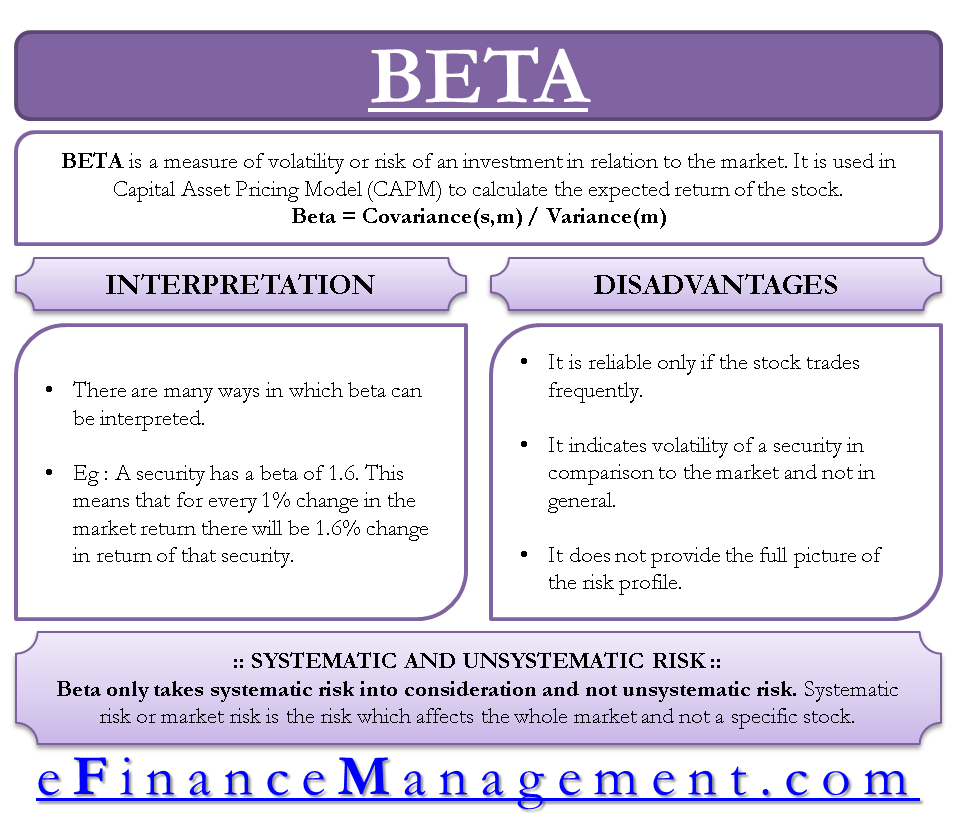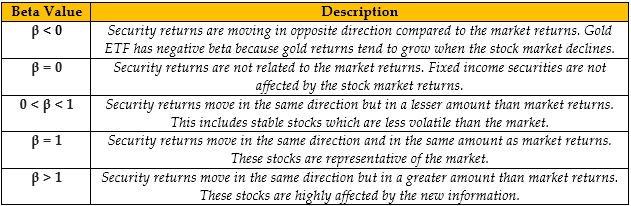# Beta

## BETA DEFINITION

Beta is a measure of volatility or risk of an investment in relation to the market. Also known as the beta coefficient (β), it is used in capital asset pricing model (CAPM) to calculate the expected return of the stock.

## FORMULA OF BETA

 Beta (β) = Covariance (ri,rm) = Correlation(ri,rm)  x Standard deviation (ri) ———————– ———————– Variance (rm) Standard deviation (rm)

ri= returns of a security, rm= market returns

Beta can be calculated by dividing the covariance between the security returns and market returns with the variance of market returns. It can also be calculated by first dividing the standard deviation of security returns with the standard deviation of market returns. The resulting value is then multiplied with the correlation of security returns and market returns.

### Covariance (ri, rm)

It measures the degree to which the returns of a security and market returns move in tandem with each other. Market returns are generally calculated from the returns of indexes like S&P 500, NASDAQ and NIFTY 50 etc. The indexes are formed in a way that they represent the whole market. If covariance is positive, market return and security return are moving together. If it is negative, both the variables are moving inversely.### Variance and Standard Deviation

Variance shows how far the values are spread from the mean. Standard deviation is the root of the variance. Standard deviation measures the risk or volatility. For example, Standard deviation of security returns measures the fluctuations of security returns around the mean of security returns.

### Correlation (ri,rm)

It measures how strong the linear relationship is between security returns and market returns. If the correlation is +1 then both the variable are moving in the same direction with a strong linear relationship. If the correlation is -1 then both the variable are moving in opposite direction with a strong linear relationship.

## SYSTEMATIC RISK AND UNSYSTEMATIC RISK

Beta only takes systematic risk into consideration. Systematic risk or market risk is the risk which affects the whole market and not a specific stock, Example- changes in interest rates, inflation, recession, war etc. This risk affects the whole economy so it cannot be avoided. It can be moderated through hedging.

Beta does not take into consideration the unsystematic risk. Unsystematic risk is the risk which specifically affects a particular company or industry and not the whole market. For example- a lawsuit is filed against a company, strike by employees in the company, poor management team etc. This risk can be avoided by investing in different stocks and diversifying your portfolio.

## INTERPRETATION OF BETA

Beta is a very convenient and easy to interpret quantitative measure. It uses regression analysis to measure the volatility. It indicates whether security is more or less risky than the overall market. There are many ways in which beta can be interpreted. For example, a security has a beta of 1.6. This means that for every 1% change in the market return there will be 1.6% change in return of that security. It can also be said that this security is 60% more risky or volatile than the overall market.The value of beta depends on the past data and period taken into consideration for calculation. The past is not always a good predictor of future. The company might change its risk profile in few years and that information will take time to reflect in company’s beta. So, the value of beta will keep changing throughout time.